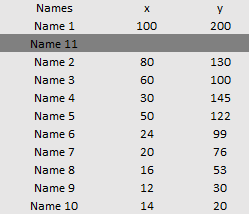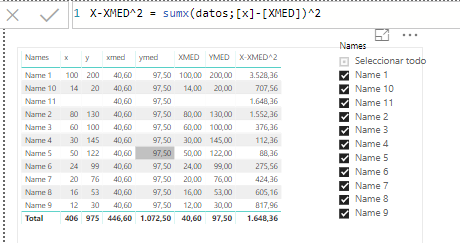cancel
Showing results for
Did you mean:

Fabric is Generally Available. Browse Fabric Presentations. Work towards your Fabric certification with the Cloud Skills Challenge.Helper I

## STEYX Calculation

Estimates, I´m becoming crazy with this simple calculation in POWER BI. The idea is to calculate the tipical error of a series of values X and Y. This is very simple in Excel with the formula STEYX but I cannot obtain the same result in Power BI, here is the formula for STEYX and the data set:(here "n" is the number of values, in this case 10 - because Name 11 doesn´t have values of X nor Y)But First things first, the calculation must be done only with the Names that has values (here Name 11 doesn´t have values, so it must not be considered in the calculation). In this example STEYX is 32.75.

When I try to do this in Power BI, I tried to separate the formula for every cumulative shown. So, the first calculation is (X-averageX)^2The values for every row are OK, but the cumulative value is not (it contain Name 11 and doesn´t sum correctly - I should have obtained 8488.4 in the total value)

So, to summarize... I want to calculate the STEYX in Power BI (formula shown above), but the calculation must use only those names that have values X and Y, and of course this calculation should change if I select diferent Names in the "Name Filter".

1 ACCEPTED SOLUTIONSolution Sage

Hi @TonyBI

you can build this modelThen try this measure:

```STEXY =
CALCULATE (
IF (
COUNTROWS ( Data ) < 3,
"Data must have at least 3 records",
VAR Y_AVG =
AVERAGE ( Data[Y] )
VAR X_AVG =
AVERAGE ( Data[X] )
VAR Y_DEV =
SUMX ( Data, POWER ( Data[Y] - Y_AVG, 2 ) )
VAR X_DEV =
SUMX ( Data, POWER ( Data[X] - X_AVG, 2 ) )
VAR XY_DEV =
POWER ( SUMX ( Data, ( Data[Y] - Y_AVG ) * ( Data[X] - X_AVG ) ), 2 )
VAR ERR_ =
SQRT (
( Y_DEV - DIVIDE ( XY_DEV, X_DEV ) )
* DIVIDE ( 1, COUNTROWS ( Data ) - 2 )
)
RETURN
ERR_
),
NOT ( ISBLANK ( Data[X] ) ),
NOT ( ISBLANK ( Data[Y] ) )
)```

Proud to be a Datanaut!3 REPLIES 3Helper I

Just another thing... this is just an example... the data set is huge, so thats why I need to be able to filter (names are related by other static atributes, like Type of Name, Region).. thx!!Solution Sage

Hi @TonyBI

you can build this modelThen try this measure:

```STEXY =
CALCULATE (
IF (
COUNTROWS ( Data ) < 3,
"Data must have at least 3 records",
VAR Y_AVG =
AVERAGE ( Data[Y] )
VAR X_AVG =
AVERAGE ( Data[X] )
VAR Y_DEV =
SUMX ( Data, POWER ( Data[Y] - Y_AVG, 2 ) )
VAR X_DEV =
SUMX ( Data, POWER ( Data[X] - X_AVG, 2 ) )
VAR XY_DEV =
POWER ( SUMX ( Data, ( Data[Y] - Y_AVG ) * ( Data[X] - X_AVG ) ), 2 )
VAR ERR_ =
SQRT (
( Y_DEV - DIVIDE ( XY_DEV, X_DEV ) )
* DIVIDE ( 1, COUNTROWS ( Data ) - 2 )
)
RETURN
ERR_
),
NOT ( ISBLANK ( Data[X] ) ),
NOT ( ISBLANK ( Data[Y] ) )
)```

Proud to be a Datanaut!Helper I

This is working perfectly! I´ll try it with the "Huge" data set, but I think It should have the same results. Thx you very much @LivioLanzo!!Announcements#### Power BI Monthly Update - November 2023

Check out the November 2023 Power BI update to learn about new features.#### Fabric Community News unified experience

Read the latest Fabric Community announcements, including updates on Power BI, Synapse, Data Factory and Data Activator.#### Exclusive opportunity for Women!

Join us for a free, hands-on Microsoft workshop led by women trainers for women where you will learn how to build a Dashboard in a Day!#### The largest Power BI and Fabric virtual conference

130+ sessions, 130+ speakers, Product managers, MVPs, and experts. All about Power BI and Fabric. Attend online or watch the recordings.Top Solution Authors
Top Kudoed Authors
Users online (2,430)